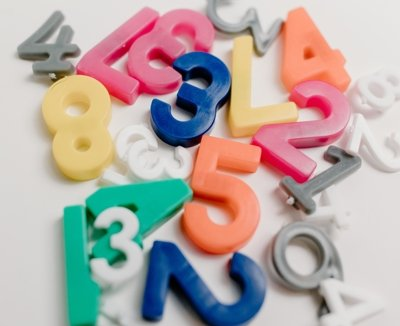Octal to Decimal conversion is the process of converting a number from the octal system (which uses eight digits: 0-7) to the decimal system (which uses ten digits: 0-9).

To do this conversion, we multiply each digit in the octal number by the corresponding power of 8 and add up the resulting products. The power of 8 starts from 0 for the rightmost digit and increases by 1 for each subsequent digit to the left.

The resulting sum is the decimal equivalent of the octal number. This conversion is important in computer programming and digital electronics where numbers are often represented in different number systems.

To convert octal to decimal:

1. Introduce the topic: Start by introducing the topic of converting octal to decimal, and ask if the other person is familiar with these number systems.
2. Explain octal and decimal: Provide a brief explanation of what octal and decimal number systems are, and how they differ from each other.
3. Discuss the conversion process: Explain how to convert an octal number to a decimal number, and provide an example to illustrate the process.
4. Ask for questions or clarifications: Ask the other person if they have any questions or if they would like you to explain any part of the process in more detail.
5. Provide practice exercises: Offer some practice exercises or problems for the other person to try on their own, and offer assistance or feedback as needed.
6. Recap and summarize: Summarize the conversation by reviewing the key points and emphasizing the importance of understanding number systems and how to convert between them.

By following these steps, you can have a productive and informative conversation about converting octal to decimal.

Exemple in Javascript code:

``````function octalToDecimal(octalNum) {
return parseInt(octalNum, 8);
}``````

Exemple in C# code:

``````public int OctalToDecimal(string octalNum)
{
int decimalNum = 0;
int power = 0;
for (int i = octalNum.Length - 1; i >= 0; i--)
{
int digit = octalNum[i] - '0';
decimalNum += digit * (int)Math.Pow(8, power);
power++;
}
return decimalNum;
}
``````

Categories:

Tags:

## Comentários

Nenhum comentário para mostrar.